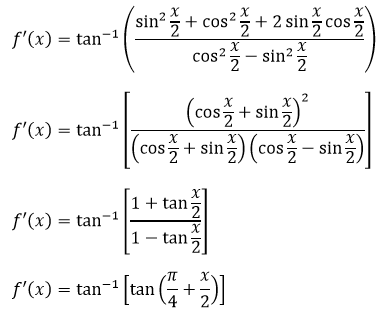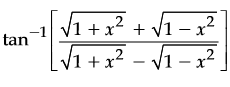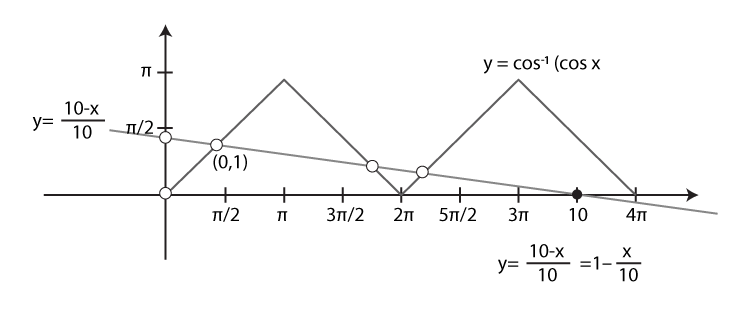# JEE Inverse Trig Functions Previous Year Questions With Solutions

Trigonometry is a measurement of a triangle and it is included with inverse functions. There are six basic inverse trigonometric functions: arcsine, arccosine, arctangent, arccotangent, arcsecant, and arccosecant. In this article, we will discuss the topic of inverse trigonometric functions along with JEE previous year problems. Students can make use of the solutions that we are offering and be one step ahead in the competition. JEE previous year questions with solutions are extremely helpful for students preparing for JEE and other competitive exams. All solutions are prepared by our subject experts to present factual information to the students related to problems covered in JEE previous exams. Students can download freely these solutions and start practicing offline.

### Properties on Inverse Trigonometric Functions

It’s important to learn the formulas well, the better you know the basic identities, the easier it will be to recognize the problem and solve them.

 Let’s have a look on some of the properties on inverse trigonometric functions: 1. sin(arcsin x) = x and arcsin(sin y) = y where -1 ≤ x ≤ 1 and -π/2 ≤ y ≤ π/2 2. tan(arctan x) = x and arctan(tan y) = y where -π/2 < y < π/2 3. sec(arcsec x) = x and arcsec(sec y) = y where, |x| ≥ 1 and 0 ≤ y < π/2 4. sin-1 (1/x) = csc-1 x 5. cos-1 (1/x) = sec-1 x 6. tan-1 (1/x) = cot-1 x 7. sin-1x + cos-1 x = π/2 8. tan-1x + cot-1x = π/2 9. sec-1 x + csc-1 x = π/2

## JEE Main Past Year Questions With Solutions on Application of Integrations

Question 1: A value of x satisfying the equation sin[cot−1(1 + x)] = cos[tan-1x], is:

(a) 1/2                            (b) -1                              (c) 0                          (d) -1/2

Solution:

Given equation is: sin[cot−1(1 + x)] = cos[tan-1x]

Let cot−1(1 + x)] = a => cot a = 1 + x …(1)

And tan-1x =b => x = tan b …(2)

Solve (1) for a in terms of sin function:

cot a = 1 + x

We know, cosec a = √(1+cot2a) = √(1 + (1 + x)2 = √(x2 + 2x + 2)

Also, sin a = 1/cosec a

=> sin a = 1/√(x2 + 2x + 2)

Or a = sin-1[1/ √(x2 + 2x + 2)]

Solve (2) for b in terms of cos function:

x = tan b

We know, sec b = √(1+tan2b) = √(1+x2)

Also, cos b = 1/sec b = 1/√(1+x2)

Or b = cos-1[1/√(1+x2)]

Given equation =>

sin(sin-1[1/ √(x2 + 2x + 2)]) = cos[cos-1(1/√(1+x2))]

=> 1/ √(x2 + 2x + 2) = 1/√(1+x2)

Squaring both sides and solving, we get

2x = -1

Or x = -1/2

Question 2: Let f(x) = (sin(tan-1x) + sin(cot-1x))2 – 1, where |x| > 1. If dy/dx = 1/2 d/dx(sin-1(f(x))) and y(√3) = π/6, then y(-√3) is equal to

(a) π/3                  (b) 2π/3                       (c) -π/6                              (d) π/7

Solution:

f(x) = (sin(tan-1x) + sin(cot-1x))2 – 1

Let tan-1(x) = A where A Є (-π/2, -π/4) U (π/4, π/2)

=> (sin(tan-1x) + sin(cot-1x))2 – 1 = (sinA + cosB)2 – 1

= 1 + 2 sin A cos A – 1

= sin 2A

= 2x/(1+x2)

Given that, dy/dx = (1/2) d/dx(sin-1(f(x)))

=> dy/dx = -1/(1+x2) for |x| > 1

(x > 1 and x< -1)

To find the value of y(-√3), integrate dy/dx. To integrate the expression, interval should be continuous. So we have to integrate the expression in both the intervals.

=>y = -tan-1x + c1 for x>1 and y = -tan-1x + c2 for x < -1

For x > 1, c1 = π/2 [because y(√3) = π/6]

But c2 can’t be determined as no other information is given for x < −1, so can’t determine the value of c2. Therefore, all the options can be true.

Question 3: If α = 3 sin-1(6/11) and β = 3 cos-1 (4/9) where the inverse trig functions take only the principal values, then the right option is

(a) cosβ > 0                 (b) cos(α + β) > 0                   (c) sin β < 0                          (d) cosα < 0

Solution:

α = 3 sin-1 (6/11) > 3 sin-1 (6/12) > 3 sin-1 (1/2) > π / 2

β = 3 cos-1 (4/9) > 3 cos-1 (4/8) > 3 cos-1 (1/2) > π

α + β > 3π/2

sin β < 0,cos α < 0,cos (α + β) > 0

Question 4: If f'(x) = tan-1(sec x + tan x), -π/2 < x < π/2, and f(0) = 0 then f(1) is equal to

(a) (π+1)/4                           (b) (π+2)/4                           (c) ¼                     (d) (π-1)/4

Solution:

f'(x) = tan-1(sec x + tan x) = tan-1(1/cosx + sin x/cos x) = tan-1[(1+sin x)/cos x]f'(x) = π/4 + x/2

=> f(x) = (π/4) x + x2/4 + c

At f(0) = 0 => c = 0

At f(1) = π/4 + 1/4 = (π+1)/4

Question 5: The value of|x| < 1/2, x ≠ 0, is equal to

(a) π/4 + (1/2) cos-1x2

(b) π/4 + cos-1x2

(c) π/4 – (1/2) cos-1x2

(d) π/4 – cos-1x2

Solution: Let x2 = cos 2A => A = (1/2) cos-1 (x2) …(1)

$tan^{-1}[\frac{\sqrt{1+cos\ 2A} +\sqrt{1-cos\ 2A}}{\sqrt{1+cos\ 2A} – \sqrt{1-cos\ 2A}}]$

= $tan^{-1}[\frac{\sqrt{2cos^2\ A} +\sqrt{2 sin^2\ A}}{\sqrt{2cos^2A} – \sqrt{2sin^2A}}]$

= $tan^{-1}[\frac{\sqrt{2}cos A +\sqrt{2}sin A}{\sqrt{2}cosA – \sqrt{2}sinA}]$

= $tan^{-1}[\frac{1+tan\ A}{1 – tan\ A}]$

We know, tan π/4 = 1, using in above equation, we get

= tan-1(tan(π/4 + A))

= π/4 + A

Using (1)

= π/4 + (1/2) cos-1(x2)

Question 6: Find the value of x satisfying the equation sin[cot-1(1+x)] = cos[tan-1x], is

(a) -1/2                        (b) -1                        (c) 0                        (d) ½

Solution:

We know, cot-1 x = sin-1 [1/√(1+x2)]  …(1)

=> $sin[sin^{-1} \frac{1}{1+(1+x)^2}] = cos(tan^{-1}x)$ …(2)

Also, we know

tan-1 x = cos-1 [1/√(1+x2)]d

(2)=> $sin[sin^{-1} \frac{1}{1+(1+x)^2}] = cos[cos^{-1} \frac{1}{\sqrt{1+x^2}}]$

or $\frac{1}{1+(1+x)^2} = \frac{1}{\sqrt{1+x^2}}$ [As sin-1 (sin A) = A Є (-π/2, π/2) and cos-1(cos A) = A Є [0, π] ]

Solving above equation, we get

1 + (1 + x)2 = 1 + x2

or x = -1/2

Question 7: The value of $cot [\sum_{n=1}^{23} cot^{-1}(1 + \sum_{k=1}^n 2k)]$ is

(a) 23/25                  (b) 25/23                    (c) 23/24                      (d) 24/23

Solution:

$cot [\sum_{n=1}^{23} cot^{-1}(1 + \sum_{k=1}^n 2k)]$

= $cot [\sum_{n=1}^{23} cot^{-1}(1 + 2 \times \frac{n(n+1)}{2}]$

= $cot [\sum_{n=1}^{23} cot^{-1}(n^2+n+1)]$

= $cot [\sum_{n=1}^{23} tan^{-1}(\frac{n+1-n}{1+n(1+n)})]$

= $cot [\sum_{n=1}^{23} tan^{-1}(n+1) – tan^{-1}n]$

= $cot [tan^{-1}(24) – tan^{-1}(1)]$

= $cot [tan^{-1} \frac{23}{25}]$

= $cot [cot^{-1} \frac{25}{23}]$

= 25/23

Question 8: Let f: [0, 4π] -> [0, π] be defined by f(x) = cos-1(cos x). The number of points x Є [0, 4π] satisfying the equation f(x) = (10-x)/10 is

(a) 1                         (b) 2                         (c) 3                     (d) None of these

Solution:

Draw graph for f(x) = cos-1(cos x) and f(x) = (10-x)/10Both the equations intersect at three different points, so the number of solutions be 3.

Question 9: Let f(x) = x cos-1(sin(-|x|)), x Є (-π/2, π/2) then which of the following is true?

(a) f′(0) = -π/2

(b) f’ is decreasing in (-π/2, 0) and increasing in (0, π/2)

(c) f is not differentiable at x = 0

(d) f’ is increasing in (-π/2, 0) and decreasing in (0, π/2)

Solution:

f(x) = x cos-1(sin(-|x|)) (Given)

=> f(x) = x cos-1(-sin(|x|))

[As sine is an odd function]

=> f(x) = x [π – cos-1(sin(|x|))

=> f(x) = x [π – (π/2 – sin-1(sin(|x|))

=> f(x) = x(π/2 + |x|)

=> $f(x) = \left\{\begin{matrix} x(\frac{\pi}{2}+x) & x \geq 0\\ x(\frac{\pi}{2}-x)& x<0 \end{matrix}\right.$

=> $f’(x) = \left\{\begin{matrix} (\frac{\pi}{2}+2x) & x \geq 0\\ (\frac{\pi}{2}-2x)& x<0 \end{matrix}\right.$

Therefore, f'(x) is decreasing (-π/2, 0) and increasing in (0, π/2).

## Trigonometry Videos

### Solving-Trigonometric-Functions### JEE TrigonometryTest your Knowledge on Inverse trig functions